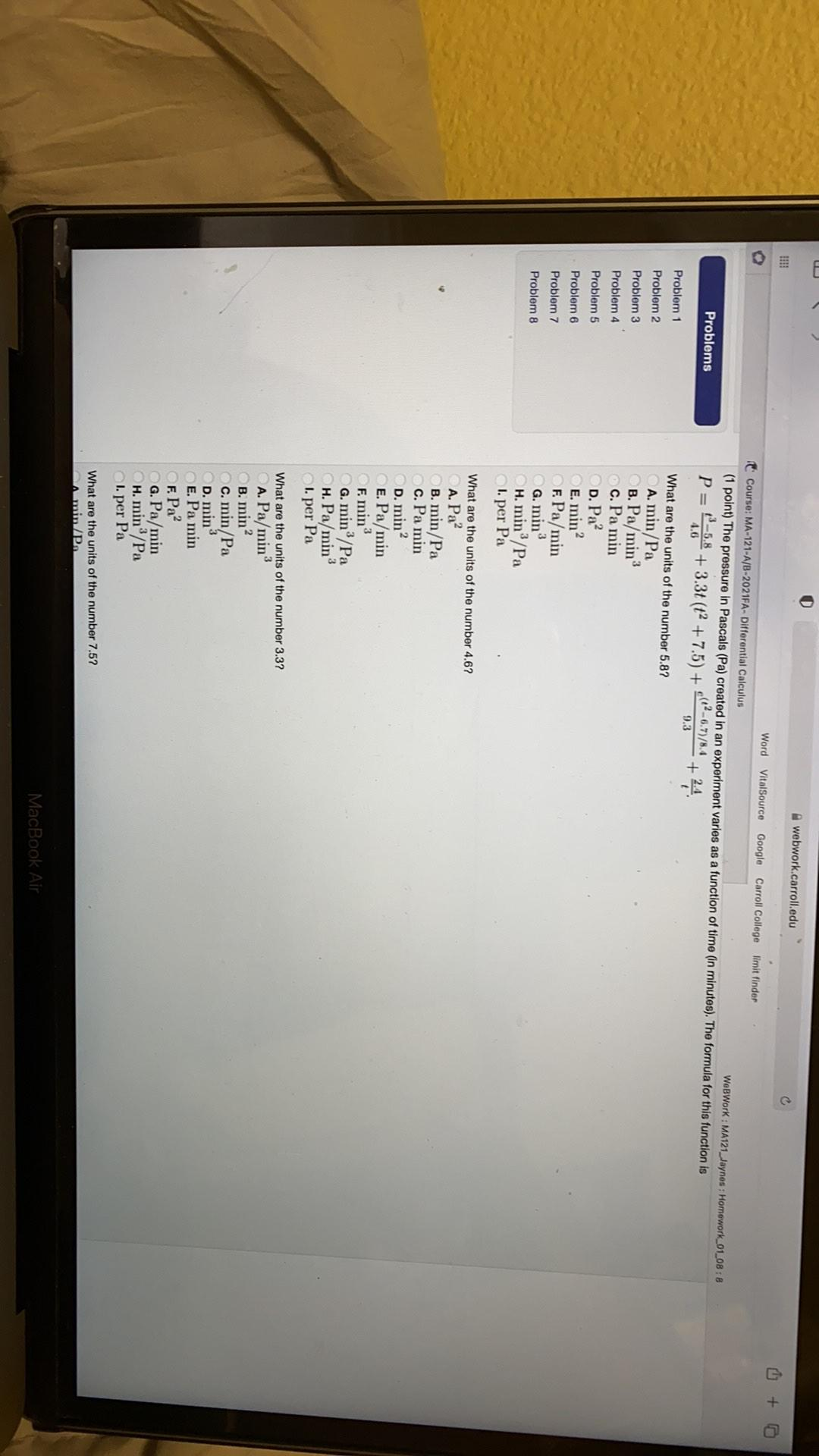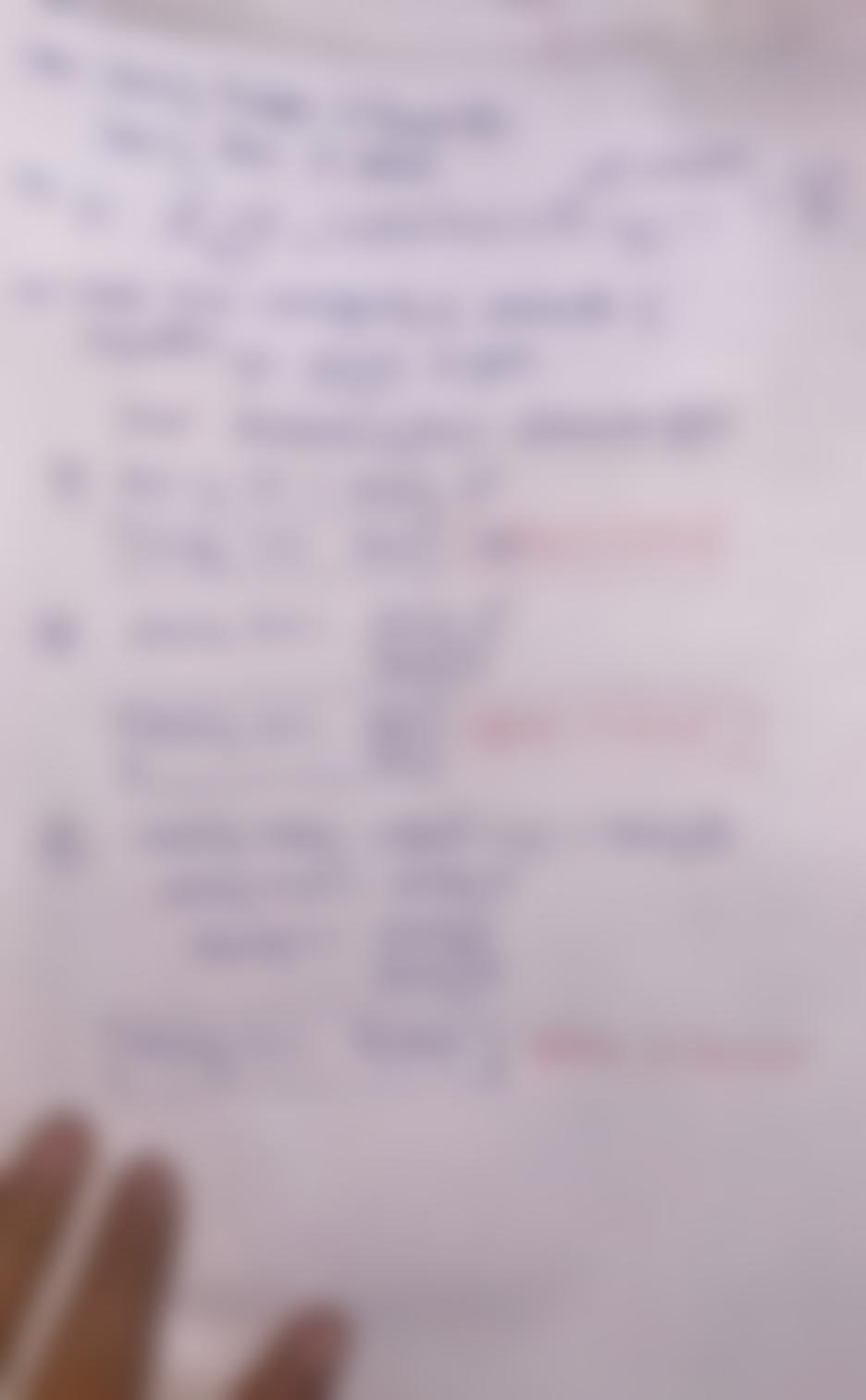Question:

webwork.carroll.edu 4 + Problems Word VitalSource Google Carroll College limit finder It Course: MA-121-A/B-2021FA-Differentialwebwork.carroll.edu 4 + Problems Word VitalSource Google Carroll College limit finder It Course: MA-121-A/B-2021FA-Differential Calculus WebWork : MA121_Jaynes : Homework_01_08:8 (1 point) The pressure in Pascals (Pa) created in an experiment varies as a function of time (in minutes). The formula for this function is P= 4.6 + 24 9.3 What are the units of the number 5.87 A. min/Pa B. Pa/min c. Pa min + 3.3t (t+ 7.5) + (12-6.7)/8.4 Problem 1 Problem 2 Problem 3 Problem 4 Problem 5 D. Pa? E. min? Problem 6 Problem 7 Problem 8 F. Pa/min G. min H. min/Pa 1. per Pa What are the units of the number 4.67 A. Pa? B. min/Pa c. Pa min D. min? E. Pa/min F. min G. min/Pa H. Pa/min I. per Pa What are the units of the number 3.37 A. Pa/min B. min? C. min/Pa D. min E. Pa min E Pa? G. Pa/min H. min/Pa I. per Pa What are the units of the number 7.5? Amin/P MacBook Air